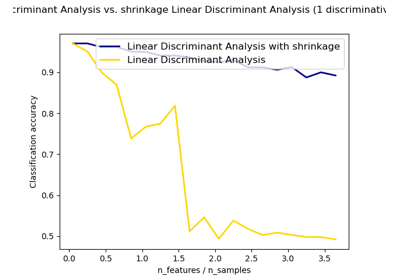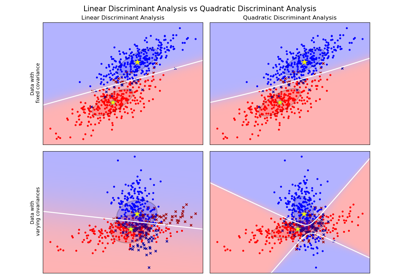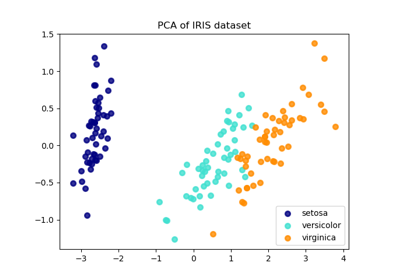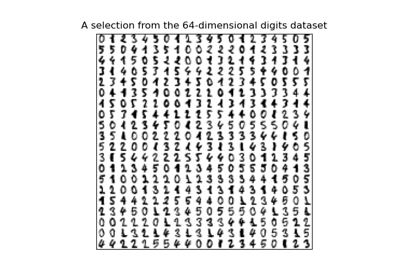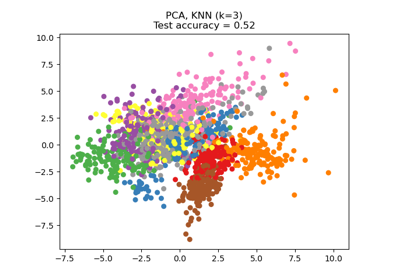# sklearn.discriminant_analysis.LinearDiscriminantAnalysis¶

class sklearn.discriminant_analysis.LinearDiscriminantAnalysis(*, solver='svd', shrinkage=None, priors=None, n_components=None, store_covariance=False, tol=0.0001)[source]

Linear Discriminant Analysis

A classifier with a linear decision boundary, generated by fitting class conditional densities to the data and using Bayes’ rule.

The model fits a Gaussian density to each class, assuming that all classes share the same covariance matrix.

The fitted model can also be used to reduce the dimensionality of the input by projecting it to the most discriminative directions, using the transform method.

New in version 0.17: LinearDiscriminantAnalysis.

Read more in the User Guide.

Parameters
solver{‘svd’, ‘lsqr’, ‘eigen’}, default=’svd’
Solver to use, possible values:
• ‘svd’: Singular value decomposition (default). Does not compute the covariance matrix, therefore this solver is recommended for data with a large number of features.

• ‘lsqr’: Least squares solution, can be combined with shrinkage.

• ‘eigen’: Eigenvalue decomposition, can be combined with shrinkage.

shrinkage‘auto’ or float, default=None
Shrinkage parameter, possible values:
• None: no shrinkage (default).

• ‘auto’: automatic shrinkage using the Ledoit-Wolf lemma.

• float between 0 and 1: fixed shrinkage parameter.

Note that shrinkage works only with ‘lsqr’ and ‘eigen’ solvers.

priorsarray-like of shape (n_classes,), default=None

The class prior probabilities. By default, the class proportions are inferred from the training data.

n_componentsint, default=None

Number of components (<= min(n_classes - 1, n_features)) for dimensionality reduction. If None, will be set to min(n_classes - 1, n_features). This parameter only affects the transform method.

store_covariancebool, default=False

If True, explicitely compute the weighted within-class covariance matrix when solver is ‘svd’. The matrix is always computed and stored for the other solvers.

New in version 0.17.

tolfloat, default=1.0e-4

Absolute threshold for a singular value of X to be considered significant, used to estimate the rank of X. Dimensions whose singular values are non-significant are discarded. Only used if solver is ‘svd’.

New in version 0.17.

Attributes
coef_ndarray of shape (n_features,) or (n_classes, n_features)

Weight vector(s).

intercept_ndarray of shape (n_classes,)

Intercept term.

covariance_array-like of shape (n_features, n_features)

Weighted within-class covariance matrix. It corresponds to sum_k prior_k * C_k where C_k is the covariance matrix of the samples in class k. The C_k are estimated using the (potentially shrunk) biased estimator of covariance. If solver is ‘svd’, only exists when store_covariance is True.

explained_variance_ratio_ndarray of shape (n_components,)

Percentage of variance explained by each of the selected components. If n_components is not set then all components are stored and the sum of explained variances is equal to 1.0. Only available when eigen or svd solver is used.

means_array-like of shape (n_classes, n_features)

Class-wise means.

priors_array-like of shape (n_classes,)

Class priors (sum to 1).

scalings_array-like of shape (rank, n_classes - 1)

Scaling of the features in the space spanned by the class centroids. Only available for ‘svd’ and ‘eigen’ solvers.

xbar_array-like of shape (n_features,)

Overall mean. Only present if solver is ‘svd’.

classes_array-like of shape (n_classes,)

Unique class labels.

Examples

>>> import numpy as np
>>> from sklearn.discriminant_analysis import LinearDiscriminantAnalysis
>>> X = np.array([[-1, -1], [-2, -1], [-3, -2], [1, 1], [2, 1], [3, 2]])
>>> y = np.array([1, 1, 1, 2, 2, 2])
>>> clf = LinearDiscriminantAnalysis()
>>> clf.fit(X, y)
LinearDiscriminantAnalysis()
>>> print(clf.predict([[-0.8, -1]]))



Methods

 Apply decision function to an array of samples. fit(X, y) Fit LinearDiscriminantAnalysis model according to the given fit_transform(X[, y]) Fit to data, then transform it. get_params([deep]) Get parameters for this estimator. Predict class labels for samples in X. Estimate log probability. Estimate probability. score(X, y[, sample_weight]) Return the mean accuracy on the given test data and labels. set_params(**params) Set the parameters of this estimator. Project data to maximize class separation.
__init__(*, solver='svd', shrinkage=None, priors=None, n_components=None, store_covariance=False, tol=0.0001)[source]

Initialize self. See help(type(self)) for accurate signature.

decision_function(X)[source]

Apply decision function to an array of samples.

The decision function is equal (up to a constant factor) to the log-posterior of the model, i.e. log p(y = k | x). In a binary classification setting this instead corresponds to the difference log p(y = 1 | x) - log p(y = 0 | x). See Mathematical formulation of the LDA and QDA classifiers.

Parameters
Xarray-like of shape (n_samples, n_features)

Array of samples (test vectors).

Returns
Cndarray of shape (n_samples,) or (n_samples, n_classes)

Decision function values related to each class, per sample. In the two-class case, the shape is (n_samples,), giving the log likelihood ratio of the positive class.

fit(X, y)[source]
Fit LinearDiscriminantAnalysis model according to the given

training data and parameters.

Changed in version 0.19: store_covariance has been moved to main constructor.

Changed in version 0.19: tol has been moved to main constructor.

Parameters
Xarray-like of shape (n_samples, n_features)

Training data.

yarray-like of shape (n_samples,)

Target values.

fit_transform(X, y=None, **fit_params)[source]

Fit to data, then transform it.

Fits transformer to X and y with optional parameters fit_params and returns a transformed version of X.

Parameters
X{array-like, sparse matrix, dataframe} of shape (n_samples, n_features)
yndarray of shape (n_samples,), default=None

Target values.

**fit_paramsdict

Returns
X_newndarray array of shape (n_samples, n_features_new)

Transformed array.

get_params(deep=True)[source]

Get parameters for this estimator.

Parameters
deepbool, default=True

If True, will return the parameters for this estimator and contained subobjects that are estimators.

Returns
paramsmapping of string to any

Parameter names mapped to their values.

predict(X)[source]

Predict class labels for samples in X.

Parameters
Xarray_like or sparse matrix, shape (n_samples, n_features)

Samples.

Returns
Carray, shape [n_samples]

Predicted class label per sample.

predict_log_proba(X)[source]

Estimate log probability.

Parameters
Xarray-like of shape (n_samples, n_features)

Input data.

Returns
Cndarray of shape (n_samples, n_classes)

Estimated log probabilities.

predict_proba(X)[source]

Estimate probability.

Parameters
Xarray-like of shape (n_samples, n_features)

Input data.

Returns
Cndarray of shape (n_samples, n_classes)

Estimated probabilities.

score(X, y, sample_weight=None)[source]

Return the mean accuracy on the given test data and labels.

In multi-label classification, this is the subset accuracy which is a harsh metric since you require for each sample that each label set be correctly predicted.

Parameters
Xarray-like of shape (n_samples, n_features)

Test samples.

yarray-like of shape (n_samples,) or (n_samples, n_outputs)

True labels for X.

sample_weightarray-like of shape (n_samples,), default=None

Sample weights.

Returns
scorefloat

Mean accuracy of self.predict(X) wrt. y.

set_params(**params)[source]

Set the parameters of this estimator.

The method works on simple estimators as well as on nested objects (such as pipelines). The latter have parameters of the form <component>__<parameter> so that it’s possible to update each component of a nested object.

Parameters
**paramsdict

Estimator parameters.

Returns
selfobject

Estimator instance.

transform(X)[source]

Project data to maximize class separation.

Parameters
Xarray-like of shape (n_samples, n_features)

Input data.

Returns
X_newndarray of shape (n_samples, n_components)

Transformed data.

## Examples using sklearn.discriminant_analysis.LinearDiscriminantAnalysis¶